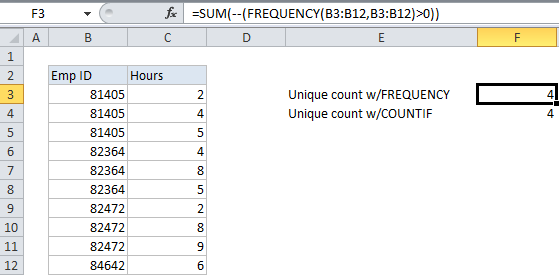## Excel Office

Excel How Tos, Tutorials, Tips & Tricks, Shortcuts

# Count unique numeric values in a range in Excel

This tutorial shows how to Count unique numeric values in a range in Excel using the example below;

## Formula

`=SUM(--(FREQUENCY(data,data)>0))`## Explanation

To count unique numeric values in a range, you can use a formula based on the FREQUENCY and SUM functions.

For example, assume you have a list of employee numbers together with hours worked on “Project X”, and you want know how many employees worked on that  project. Looking at the data, you can see that the same employee numbers appear more than once, so what you want is a count of the unique employee numbers that appear in the list.

Worked Example:   Excel Frequency Function Example

The employee numbers appear in the range B3:B12. The formula in F3 is:

`=SUM(--(FREQUENCY(B3:B12,B3:B12)>0))`

### How this formula works

The FREQUENCY function returns an array of values that correspond to “bins”. In this case, we supply the same set of numbers for both the data array and bins array.The result is that FREQUENCY returns an array of values that represent a count for each numeric values in the data array. This works because FREQUENCY has a special feature that automatically returns zero for any numbers that have already appeared in the data array. The return array looks like this:

`{3;0;0;3;0;0;3;0;0;1;0}`

Next, each of these values is tested to be greater than zero. The result looks is an array like this:

`{TRUE;FALSE;FALSE;TRUE;FALSE;FALSE;TRUE;FALSE;FALSE;TRUE;FALSE}`

Now each TRUE in the list represents a unique number in the list, and we just need to add up the TRUE values with SUM.

Worked Example:   Count unique numeric values with criteria in Excel

SUM won’t add logical values, so we first coerce the logicals to 1 or 0 with a double hyphen to get:

`{1;0;0;1;0;0;1;0;0;1;0}`

Finally, SUM adds these values up and returns the total, which in this case is 4.

Worked Example:   Count unique text values with criteria

Note: you could also use SUMPRODUCT to sum the items in the array.

### Using COUNTIF instead of FREQUENCY to count unique values

Another way to count unique numeric values is to use COUNTIF instead of FREQUENCY. This is a much simpler formula, but beware that using COUNTIF on larger data sets to count unique values can cause performance issues. The FREQUENCY-based formula, while more complicated, calculates much faster.